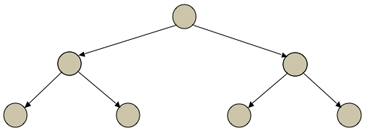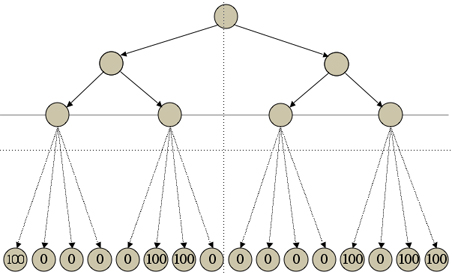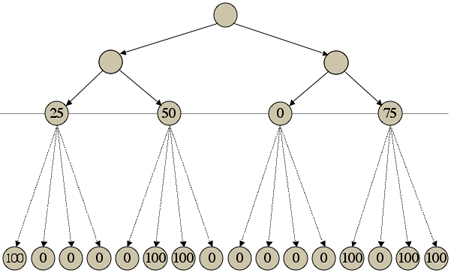C H A P T E R  8
 Probabilistic Search

### 8.1 Introduction

In the preceding chapter, we examined various approaches to incomplete search of game trees. In each approach, the evaluation of states is based on local properties of those states (i.e. properties that do not depend on the game tree as a whole). In many games, there is no correlation between these local properties and the likelihood of success in completing a game successfully.

In this chapter, we look at some alternative methods based on probabilistic analysis of game trees. In the next section, we examine an approach based on Monte Carlo game simulation. In the subsequent section, we look at a more sophisticated variation called Monte Carlo Tree Search.

### 8.2 Monte Carlo Search

The basic idea of Monte Carlo Search (MCS) is simple. In order to estimate the value of a non-terminal state, we make some probes from that state to the end of the game by selecting random moves for the players. We sum up the total reward for all such probes and divide by the number of probes to obtain an estimated utility for that state. We can then use these expected utilities in comparing states and selecting actions.

Monte Carlo can be used in compulsive deliberation fashion to evaluate the immediate successors of the current state. However, it can also be used as an evaluation function for heuristic search. The former approach can then be seen as a special case of the latter where the depth limit for expansion is set to 0. In the latter case, search takes the form of two phases of search - the expansion phase and the probe phase.

The expansion phase of Monte Carlo is the same as bounded depth heuristic search. The tree is explored until some fixed depth is reached. The tree shown below illustrates this process.The probe phase of Monte Carlo takes the form of exploration from each of the fringe states reached in the expansion phase, for each making random probes from there to a terminal state. See below.The values produced by each probe are added up and divided by the number of probes for each state to obtain an expected utility for that state. These expected utilities are then compared to determine the relative utilities of the fringe states produced at the end of the expansion phase.The following procedure is a simple implementation of the two-phase Monte Carlo method, using 4 probes per state. The implementation is similar to that of the heuristic search player introduced in the preceding chapter. The main difference is that the Monte Carlo method is used to evaluate states rather than general heuristics.

 ```function maxscore (role,state,level) {if (findterminalp(state,game)) {return findreward(role,state,game)}; if (level>levels) {return montecarlo(role,state,4)}; var actions = findlegals(role,state,game); var score = 0; for (var i=0; iscore) {score = result}}; return score} function montecarlo (role,state,count) {var total = 0; for (var i=0; i

Note that, in the probe phase of Monte Carlo, only one action is considered for each player on each step. Also, there is no additional processing (e.g. checking whether states have been previously expanded). Consequently, making probes in Monte Carlo is fast, and this enables players to make many such probes.

One downside on the Monte Carlo method is that it can be optimistic. It assumes the other players are playing randomly when in fact it is possible that they know exactly what they are doing. It does not help if most of the probes from a position in Chess lead to success if one leads to a state in which one's player is checkmated and the the other player sees this. This issue is addressed to some extent in the MCTS method described below.

Another drawback of the Monte Carlo method is that it does not take into account the structure of a game. For example, it may not recognize symmetries or independences that could substantially decrease the cost of search. For that matter, it does not even recognize boards or pieces or piece count or any other features that might form the basis of game-specific heuristics. These issues are discussed further in the chapters to follow.

Even with those drawbacks, the Monte Carlo method is quite powerful. Prior to its use, general game players were at best interesting novelties. Once players started using Monte Carlo, the improvement in game play was dramatic. Suddenly, automated general game players began to perform at a high level. Using a variation of this technique, CadiaPlayer won the International General Game Playing competition 3 times. Almost every general game playing program today includes some version of Monte Carlo.

### 8.3 Monte Carlo Tree Search

Monte Carlo Tree Search (MCTS) is a variation of Monte Carlo Search. Both methods build up a game tree incrementally and both rely on random simulation of games; but they differ on the way the tree is expanded. MCS uniformly expands the partial game tree during its expansion phase and then simulates games starting at states on the fringe of the expanded tree. MCTS uses a more sophisticated approach in which the processes of expansion and simulation are interleaved.

MCTS processes the game tree in cycles of four steps each. After each cycle is complete, it repeats these steps so long as there is time remaining, at which point it selects an action based on the statistics it has accumulated to that point.

Selection. In the selection step, the player traverses the tree produced thus far to select an unexpanded node of the tree, making choices based on visit counts and utilities stored on nodes in the tree.

Expansion. The successors of the state chosen during the selection phase are added to the tree.

Simulation. The player simulates the game starting at the node chosen during the selection phase. In so doing, it chooses actions at random until a terminal state is encountered.

Backpropagation. Finally, the value of the terminal state is propagated back along the path to the root node and the visit counts and utilities are updated accordingly.

An implementation of the MCTS selection procedure is shown below. If the initial state has not been seen (i.e. it has 0 visits), then it is selected. Otherwise, the procedure searches the successors of the node. If any have not been seen, then one of the unseen nodes is selected. If all of the successors have been seen before, then the procedure uses the selectfn subroutine (described below) to find values for those nodes and chooses the one that maximizes this value.

 ```function select (node) {if (node.visits==0) {return node}; for (var i=0; iscore) {score = newscore; result=node.children[i]}}; return select(result)} ```

One of the most common ways of implementing selectfn is UCT (Upper Confidence bounds applied to Trees). A typical UCT formula is vi + c*sqrt(log np / ni). vi here is the average reward for that state. c is an arbitrary constant. np is the total number of times the state's parent was picked. ni is the number of times this particular state was picked.

 ```function selectfn(node) {return node.utility/node.visits+Math.sqrt(2*Math.log(node.parent.visits)/node.visits)} ```

Of course, there are other ways that one can evaluate states. The formula here is based on a combination of exploitation and exploration. Exploitation here means the use of results on previously explored states (the first term). Exploration means expansion of as-yet unexplored states (the second term).

Expansion in MCTS is basically the same as that for MCS. An implementation for a single player game is shown below.

 ```function expand (node) {var actions = findlegals(role,node.state,game); for (var i=0; i

On large games with large time bounds, it is possible that the space consumed in this process could exceed the memory available to a player. In such cases, it is common to use a variation of the selection procedure in which no additional states are added to the tree. Instead, the player continues doing simulations and updating its numbers for already-known states.

Simulation for MCTS is essentially the same as simulation for MCS. So the same procedure can be used for both methods.

Backpropagation is easy. At the selected node, the method records a visit count and a utility. The visit count in this case is 1 since it was a newly processed state. The utility is the result of the simulation. The procedure then propagates to ancestors of this node. In the case of a single player game, the procedure adds 1 to the visit count of each ancestor and augments its total utility by the utility obtained on the latest simulation. See below. In the case of a multiple-player game the propagated value is the minimum of the values for all opponent actions.

 ```function backpropagate (node,score) {node.visits = node.visits+1; node.utility = node.utility+score; if (node.parent) {backpropagate(node.parent,score)}; return true} ```

Expanding the method to multiple-player games is tedious but poses no significant problems. Max nodes and min nodes must be differentiated. Expansion must create a bipartite tree, alternating between max nodes and min nodes. And backpropagation must be adjusted accordingly.